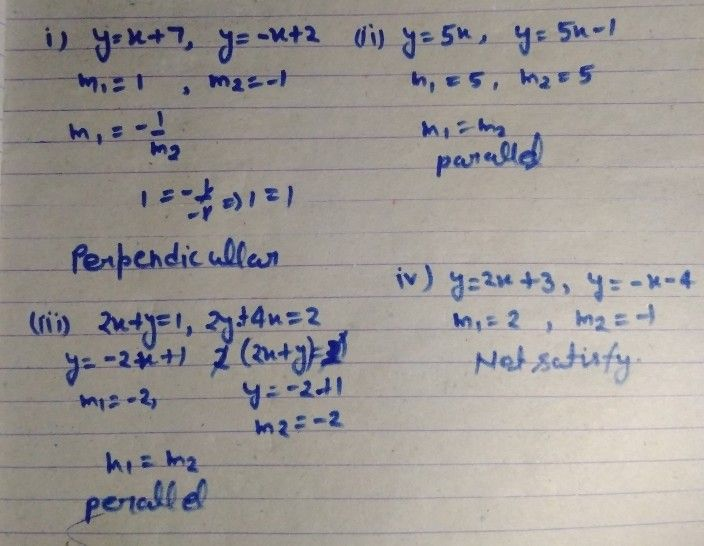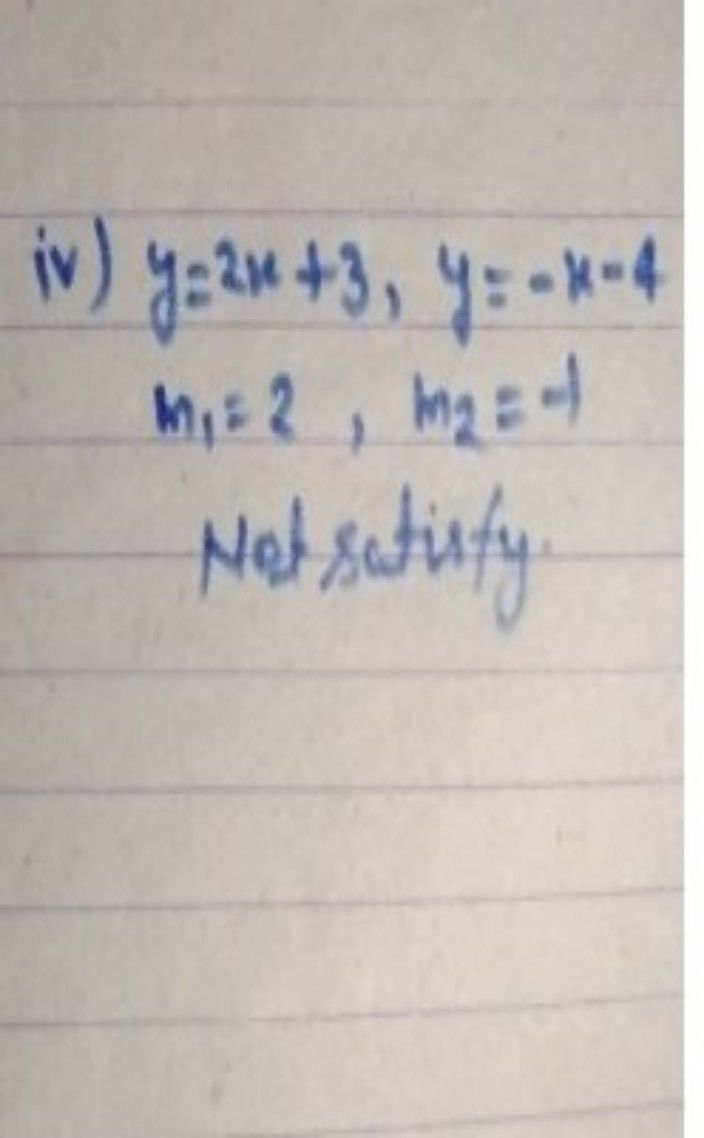Symbol
ProblemLearning Task $2$ Without graphing, tell whether the graphs of the system of $quations$ are parallel, perpendicular, intersecting or coinciding. Do it in your otebook. $1.y=x+7$ and $y=-x+2$ $3.2x+y=1$ and $2y+4x=2$ $2.Y=5x$ and $y=5x-1$ $4.X=2x+3$ and $y=-x-4$ $3$
7th-9th grade
Algebra
Search count: 112
SolutionQanda teacher - Vineetaplease give me review by 5 ?Student
where is numberiv.?
iv.?Qanda teacher - VineetaokStudent
why there is not parallel or intersecting or even perpendicular why net satisfy? whta isa the answer?Qanda teacher - Vineeta
because m1 is not equal m2
or not equal -1/m2Student
so what is tge answer perpendicular or parallel or interswcting?Qanda teacher - Vineeta
waitStudent
please faster
where?Qanda teacher - Vineeta
ok
intercecting
please give me review by 5 ?Student
ok